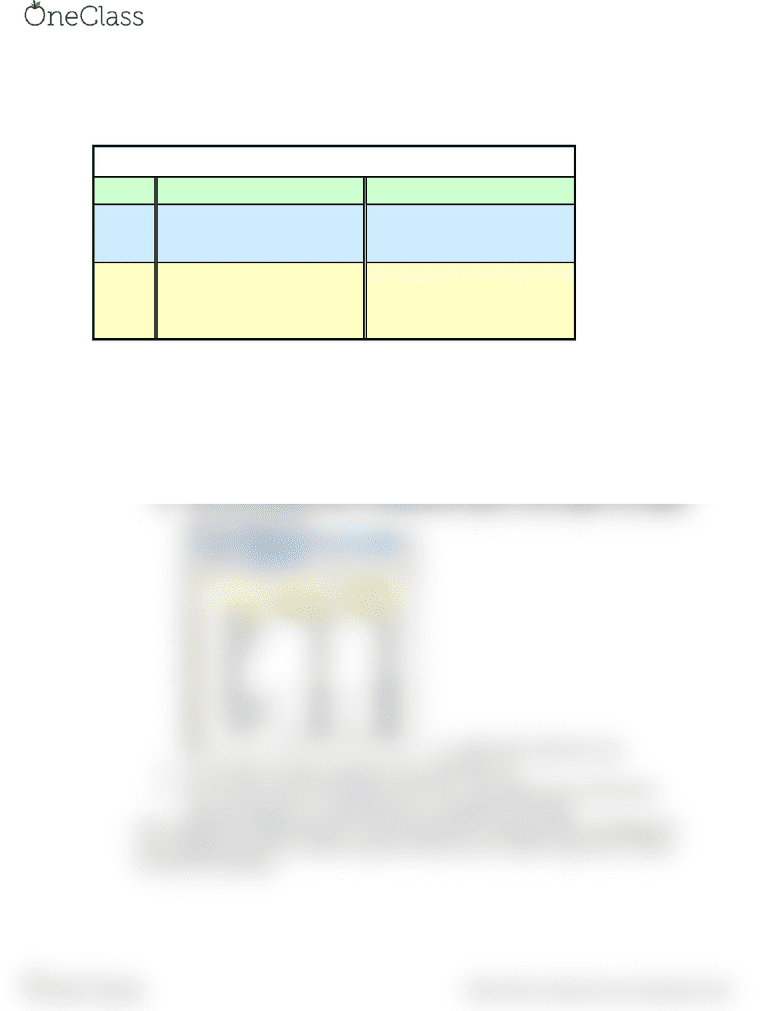Study Guides (380,000)
CA (150,000)
U of G (7,000)
ACCT (100)
Final

ACCT 2230 Study Guide - Final Guide: Fixed Cost, Variable Cost, Income Statement

Department
Accounting
Course Code
ACCT 2230
Professor
Alireza Talebi
Study Guide
Final

This preview shows half of the first page. to view the full 2 pages of the document.Chapter 3
- Types of cost Behaviours
Summary of Variable and Fixed Cost Behaviour
Cost In Total Per Unit
Variable Total variable cost is Variable cost per unit remains
proportional to the activity the same over wide ranges
level within the relevant range. of activity.
Total fixed cost remains the
same even when the activity Fixed cost per unit goes
Fixed level changes within the down as activity level goes up.
relevant range.
- Mixed costs have both fixed and variable components
- Mixed cost equation is Y=a +bX
Y= the total mixed cost
A= the total fixed cost (the vertical intercept of the line)
B= the variable cost per unit of the activity (the slope of the line)
X= the activity level of activity
- High Low Method
The total variable per hour of maintenance is equal to the change in cost divided
by the change hours
so, 2400/ 300= \$8.00 per hour
You can then use this to solve for your total fixed cost
You can then use the total fixed cost for the cost equation for maintenance
The cost equation is y= total fixed cost + (variable per hour)(X)
- The contribution margin format for income statement is mainly used for management
thus is different from the tradition approach because the tradition approach is mainly
for external reporting
find more resources at oneclass.com
find more resources at oneclass.com